# What is impedance in resistance welding?

Impedance (Z) is the total opposition to current flow. It is made up of the Resistance (R) and Reactance (X).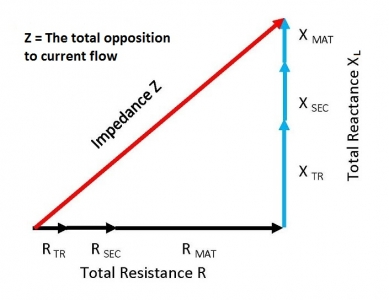The formula for Impedance is: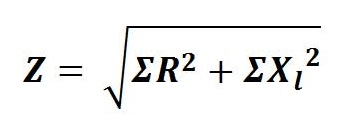Reactance (X) is only present in AC circuits.
Reactance (X) is created by the induced magnetic field around the conductors in the secondary.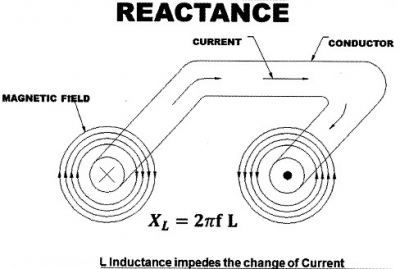This induced field wants to stay in a steady state but in AC, current is constantly changing (reversing from positive to negative) with the AC sine wave. The Reactance builds momentum in each half cycle and resists changing to the other direction. The stronger the magnetic field the larger the reactance. The size of the welder throat is a factor in determining this magnetic strength. Larger throat areas create stronger magnetic fields.Larger K & L lead to increased reactance.

Additionally magnetic material in the form of tooling or the workpiece itself in the throat adds to the reactance and reduces the current flow.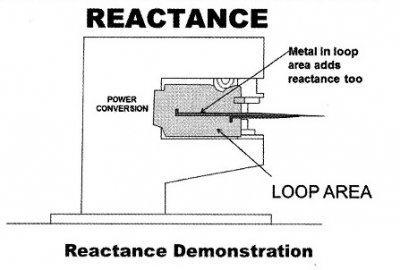Magnetic tooling or the workpiece in the throat of an AC Resistance Welder

Now look at the Resistance in the welding circuit. The transformer secondary and terminals, secondary conductors in the loop area, electrodes, electrode holders and the workpiece are all components with resistances (R) to current flow. In addition to these conductors each electrical connection has a resistance. The resistances of the conductors and connections in the secondary have to be combined for the total resistance in the system (R).
Impedance (Z) is the square root of the sum of the total resistance (R) and the total reactance (X) squared.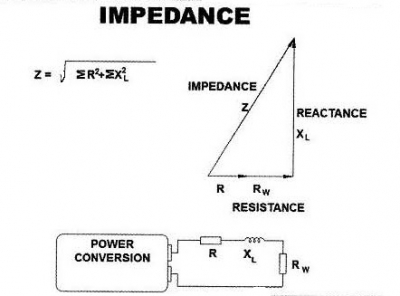Impedance (Z) is the square root of the sum of the total resistance (R) and the total reactance (X) squared

Lowering impedance will optimize current flow. To accomplish this minimize the throat size. Whenever possible, run insulated conductors and cables close together. Minimize the number of connections. Keep connections clean and tight. Remove magnetic material from in or around the throat area.

Impedance is an inherent factor of AC equipment. DC and MFDC do not have any reactance or magnetic material issues. Therefore reactance is eliminated and only resistance is a factor. Losses are reduced substantially.
Impedance in a DC/MFDC circuit is:Reference: RWMA Resistance Welding Manual Revised 4th Ed Chapter 19

Roman Manufacturing Inc.

### Have a Question?

Do you have a question that is not covered in our knowledgebase? Do you have questions regarding the above article? Click here to ask the professor.